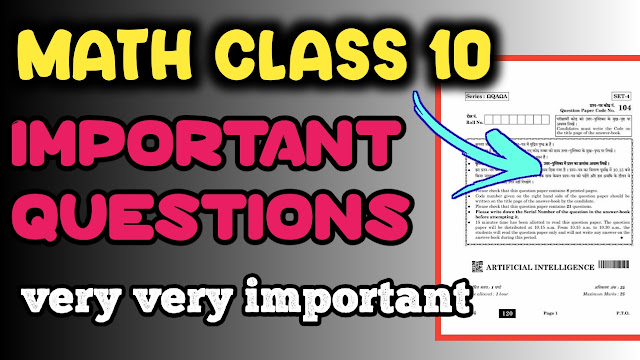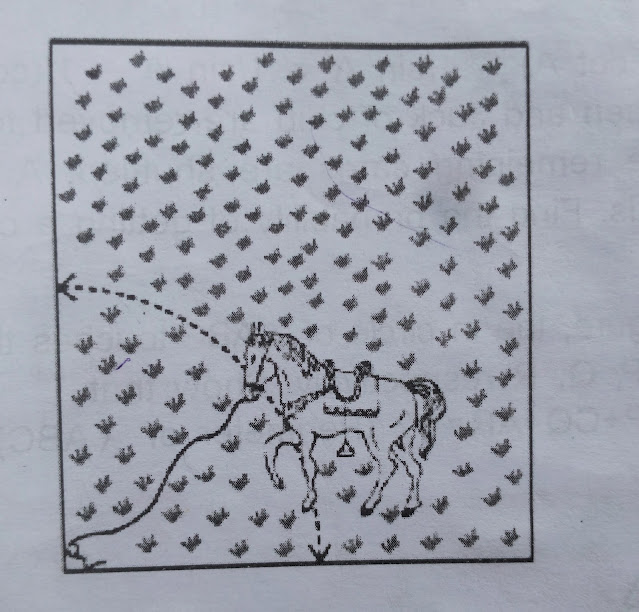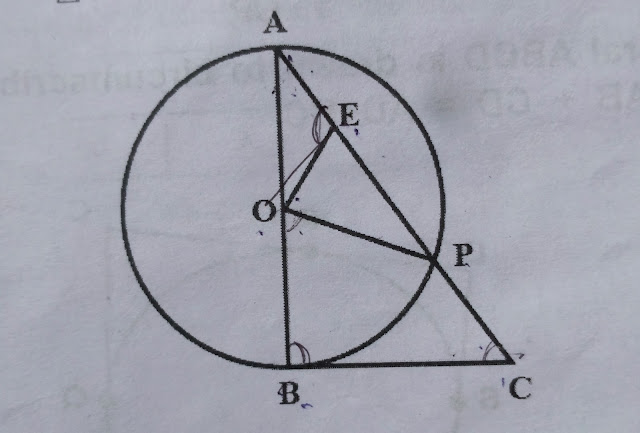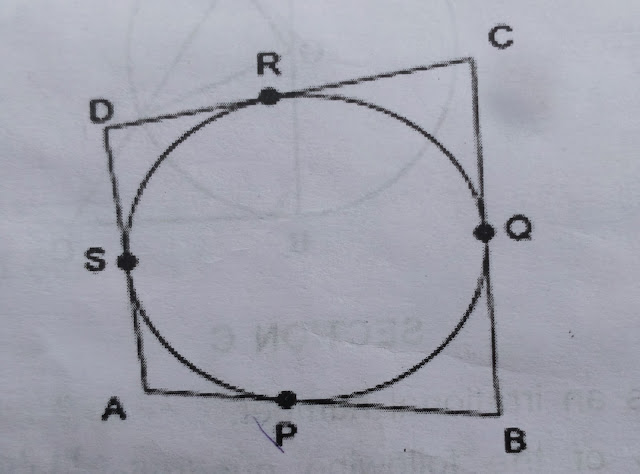# Math class 10 most important questions for board exam 2023

Hello students, welcome to our new article, in this article we are going to so you math class 10 important questions. So, let's start the article.Math class 10 important questions 2023

### Math class 10 important questions

Here are some important questions of math class 10 given below.

#### Math class 10 important questions (Section A):-

1. If two positive integers a and b are written as a=x3y2 and b=xy3 , where x,y are prime numbers, then HCF of (a,b) is
A. xy
B. xy2
C.x3y2
D. xy3

2. The largest number which divides 70 and 125 , leaving reminders 5 and 8 respectively is-
A.13
B. 65
C. 875
D. 620

3. The product of two consecutive natural numbers is always-
A. Prime number
B. Even number
C. Odd number
D. Even or Odd

4. The pair of equations x+2y+5=0 and -3x-6y+1=0 has ?
A. A unique solution
B. Exactly two solution
C. Infinite many solution
D. No solution

5. A quadratic polynomial whose one zero is 6 and some of zeroes is 0, is__
A. x2-6x+2
B. x2-36
C. x2-6
D. x2-3

6. In ∆ABC and ∆ DEF, angle B= angle E, angle F= angle C, and AB= 3DE. Then , the two triangles are__
A. Congruent but not similar
B. Similar but not congruent
C. Neither congruent nor similar
D. Congruent as well as similar

7. It is given that ∆ABC~∆DEF, angle A=30°, angle C=50°, AB=5cm, AC=8cm and DF=7.5 cm. Then which of the following is true?
A. DE=12cm, angle F=50°
B. DE=12 cm, angle F= 100°
C. EF= 12 cm , angle D = 100°
D. EF= 12 cm, angle D=50°

8. If (a/3, 4) is mid point of the segment joining the points P(-6,5) and R(-2,3) then the value of "a" is?
A. 12
B. 6
C. -12
D. -4

9. sinθ+ sin2θ =1 then cos2θ+cos4θ=?
A. -1
B. 0
C. 1
D. 2

10. 5tan2A- 5 sec2A+1 is equal to
A. 6
B. -5
C. 1
D. -4

11. If x tan45° sin30°=cos30°tan30°, then the value of x is?
A. √3
B. 12
C. 0
D. 1

12. The distance of point P(2,3) from x-axis is ?
A. 2
B. 3
C. 1
D. 5

13. The length of an arc of a circle with radius 12 cm is 4πcm , then the central angle of this arc is ?
A. 120°
B. 75°
C. 60°
D. 150°

14. The radius of a circle whose circumference is equal to the sum of the circumference of the two circles of diameter 36cm and 20cm is_
A. 56cm
B. 42cm
C. 28 cm
D. 16cm

15. a cylinder, A con and hemisphere are of equal base and have the same height (equal to radius).
What is the ratio of their volumes?
A. 3:1:2
B. 3:2:1
C. 1:2:3
D. 1:3:2

16. in the following figure if O is the centre of a circle, PQ is a chord and the tangent PR at P,  makes an angle of 50° with PQ then triangle POQ is equal to_

A. 100°
B. 80°
C. 90°
D. 75°

17. For the following distribution
Class 10 most important questions
Class interval frequency
0-10 20
10-20 30
20-30 24
30-40 40
40-50 18

The sum of lower limits of the modal class and the median is ?
A. 20
B. 30
C. 40
D. 50

18. Mode and mean of a data are 12k and 15k . Median of the data is_
A. 12k
B. 14k
C. 15k
D. 16k

19. a man has some hens and cows. If the numbers of heads be 48 and the number of feet be 140 how many cows are there?

20. In the figure, if ∆ABE≅∆ACD , show that ∆ADE~ ∆ABC.

21. If tanA=3/4, find sinAcosA

22. Find the area of a sector of a circle, given that angle of the sector is 120° and the radius of the circle is 21cm.

23. A hoser is tied to a peg at one corner of a square shaped grass field of side 15 m by means of a 5m long rope(see figure).Class 10 math important questions

Find the are of that part of the field in which the horse can graze(use π=3.14)

24. In the given figure, BC is a tangent to the circle with center O. OE bisects AP. Prove that ∆AEO~∆ABC.Important questions class 10 maths

25. Prove that √5 is an irrational number.

26. find the zeros of the following quadratic polynomial and verify the relationship between the zeros and the conficients.
x2+2x-15

27. The coach of a cricket team buys 7 bats and 6 balls for RS 3800. Later , he buys 3 bats and 5 balls for Rs1750. Find the cost of each bat and each ball.

28. Draw the graph of a linear equations x-y+2=0 and 4x-y-4=0 , hence find the solution.

29. Prove that:
1/(cosecA-cotA)-1/sinA=1/sinA-1/(cosec A+cotA)

30. The king and jack of club are removed from a deck of 52 playing cards and the remaining cards are suffeled.
A card is drawn from the remaining cards.

Find the probability of a getting card of
A. Heart
B. Queen
C. Club

31. A quadrilateral ABCD is drawn to circumscribe a circle , prove that AB+CD=AD+BC.Maths important questions class 10

32. A train travels at a certain average speed for a distance of 63 km and then travels a distance of 72 km at an average speed of 6km/he more than its original speed. If it takes 3 hours to complete the total journey, what is it's original average speed?

33. State the basic proportionality theorem and use this theorem to find AD if ABCDis a trapezium in which AB||DC and P, Q are points on AD and BC respectively, such that PQ|| DC if PD=18cm, BQ=35cm and QC=15cm.

34. A solid wooden you is in the shape of a right circular cone mounted on a hemisphere with same radius. If the radius of the hemisphere is 4.2 cm and the total height of the toy is 10.2 cm, find the volume of the wooden toy.

35. Tent is in the shape of a cylinder surmounted by conical top. If the height and diameter of the cylindrical part are 2.1m and 4m respectively and the slant height of the top is 2.8 m. Find the area of the canvas used for making the tent, also find the cost of canvas of the tent at the rate of Rs500 per meter square.

36. If the median of the given data is 46 and sum of the frequency is 230,
Find
A.  x and y
B. Hence find the mode of complete distribution

Class 10 most important questions
Class interval frequency
10-20 12
20-30 30
30-40 x
40-50 65
50-60 y
60-70 25
70-80 18